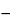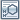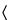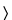Sumatriptan Tablets
DEFINITION
Sumatriptan Tablets contain NLT 90.0% and NMT 110.0% of the labeled amount of sumatriptan (C14H21N3O2S).
IDENTIFICATION
The IR spectrum exhibits main bands at or near (±1) wave numbers (cm1) 1708, 1567, 1339, 1300, 1235, 1207, 989, and 844.
•  B. The retention time of the major peak of the Sample solution corresponds to that of the Standard solution, as obtained in the Assay.
ASSAY
•  Procedure
Buffer solution A:  2.93 g/L of monobasic sodium phosphate, 1.3 mL/L of dibutylamine, and 0.4 mL/L of phosphoric acid in water. Adjust with 10 N sodium hydroxide to a pH of 6.5.
Buffer solution B:  3.9 g/L of monobasic sodium phosphate. Adjust with 10 N sodium hydroxide to a pH of 6.5 before dilution.
Mobile phase:  Acetonitrile and Buffer solution A (1:3)
Diluent:  Acetonitrile and Buffer solution B (1:3)
Standard solution:  Equivalent to 0.1 mg/mL of sumatriptan from USP Sumatriptan Succinate RS in Diluent
Sample solution:  Nominally 0.1 mg/mL of sumatriptan in Diluent. [Note—Sonicate if necessary. ]
Chromatographic system
Mode:  LC
Detector:  UV 282 nm
Column:  4.6-mm × 25-cm; 5-µm packing L1
Flow rate:  1.5 mL/min
Injection size:  10 µL
Run time:  10 min
System suitability
Sample:  Standard solution
Suitability requirements
Tailing factor:  NMT 2.0
Relative standard deviation:  NMT 1.0%
Analysis
Samples:  Standard solution and Sample solution
Calculate the percentage of the labeled amount of sumatriptan (C14H21N3O2S) in the portion of Tablets taken:
Result = (rU/rS) × (CS/CU) × (Mr1/Mr2) × 100
 rU = = peak response from the Sample solution rS = = peak response from the Standard solution CS = = concentration of USP Sumatriptan Succinate RS in the Standard solution (mg/mL) CU = = nominal concentration of the Sample solution (mg/mL) Mr1 = = molecular weight of sumatriptan, 295.4 Mr2 = = molecular weight of sumatriptan succinate, 413.5
Acceptance criteria:  90.0%–110.0% of the labeled amount of sumatriptan (C14H21N3O2S).
PERFORMANCE TESTS
Test 1
Medium:  0.01 N hydrochloric acid; 900 mL
Apparatus 2:  30 rpm
Time:  15 min
Standard solution:  0.025 mg/mL of USP Sumatriptan Succinate RS in Medium
Sample solution:  Pass a portion of the solution under test through a suitable filter of 0.45-µm pore size. Dilute with Medium if necessary.
Instrumental conditions
Mode:  Spectrophotometry
Detector:  UV 282 nm
Blank:  Medium
Analysis
Samples:  Standard solution and Sample solution
Calculate the percentage of the labeled amount of sumatriptan (C14H21N3O2S) dissolved:
Result = (AU/AS) × (CS/L) × D × V × (Mr1/Mr2) × 100
 AU = = absorbance of the Sample solution AS = = absorbance of the Standard solution CS = = concentration of the Standard solution (mg/mL) L = = label claim (mg/Tablet) D = = dilution factor of the Sample solution V = = volume of Medium, 900 mL Mr1 = = molecular weight of sumatriptan, 295.4 Mr2 = = molecular weight of sumatriptan succinate, 413.5
Tolerances:  NLT 80% (Q) of the labeled amount of sumatriptan is dissolved.
Test 2:  If the product complies with this test, the labeling indicates that it meets USP Dissolution Test 2.
Medium:  0.01 N hydrochloric acid; 900 mL
Apparatus 2:  50 rpm
Time:  15 min
Standard solution:  (L/900) mg/mL of USP Sumatriptan Succinate RS in Medium, where L is the Tablet label claim in mg
Sample solution:  Pass a portion of the solution under test through a suitable filter of 0.45-µm pore size.
Instrumental conditions
Mode:  Spectrophotometry
Detector:  UV 282 nm
Cell path length:  1 cm
Blank:  Medium
Analysis
Samples:  Standard solution and Sample solution
Calculate the percentage of the labeled amount of sumatriptan (C14H21N3O2S) dissolved:
Result = (AU/AS) × (CS/L) × V × (Mr1/Mr2) × 100
 AU = = absorbance of the Sample solution AS = = absorbance of the Standard solution CS = = concentration of the Standard solution (mg/mL) L = = label claim (mg/Tablet) V = = volume of Medium, 900 mL Mr1 = = molecular weight of sumatriptan, 295.4 Mr2 = = molecular weight of sumatriptan succinate, 413.5
Tolerances:  NLT 80% (Q) of the labeled amount of sumatriptan is dissolved.
•  Packaging and Storage: Preserve in well-closed containers. Store between 2and 30.
•  Labeling: When more than one Dissolution test is given, the labeling states the Dissolution test used only if Test 1 is not used.
USP Sumatriptan Succinate RS1H-Indole-5-methanesulfonamide, 3-[2-(dimethylamino)ethyl]-N-methyl-, butanedioate (1:1).
C14H21N3O2S·C4H6O4        413.49
Auxiliary Information— Please check for your question in the FAQs before contacting USP.
Topic/Question Contact Expert Committee
Monograph Hariram Ramanathan, M.S.
Associate Scientific Liaison
1-301-816-8313
(SM42010) Monographs - Small Molecules 4711Margareth R.C. Marques, Ph.D.
Senior Scientific Liaison
1-301-816-8106
(GCDF2010) General Chapters - Dosage Forms
Reference Standards RS Technical Services
1-301-816-8129
rstech@usp.org
USP35–NF30 Page 4732
Pharmacopeial Forum: Volume No. 35(4) Page 871